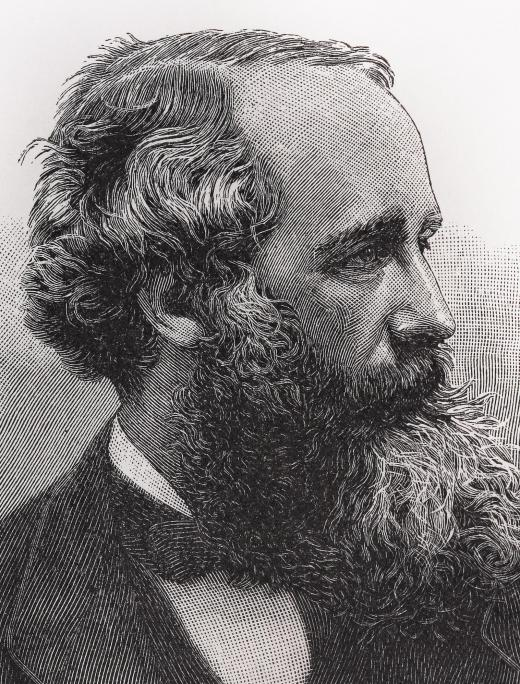Science
Fact Checked

# What is Vector Physics?

John Kinsellagh
John Kinsellagh

A vector is a visual representation of a physical quantity that has both magnitude and direction. Vector physics is the study of the various forces that act to change the direction and speed of a body in motion. The mathematical tools of vector analysis provide a useful means by which to observe the interplay of natural forces in the physical universe and to predict the impact these forces will have on matter in motion.

Vectors are symbolized by arrows of varying lengths. The relative length of each arrow represents its magnitude, which can be velocity or any other force that is capable of measurement. Each arrow has a certain direction, which is denoted on a Cartesian plane using the geographic axes of North, South, East, and West. The tail of each vector starts from the Cartesian coordinates of (0,0) and the position of the head or arrow denotes its respective direction.Vector analysis plays a prominent role in electromagnetism, which was discovered by James Clerk Maxwell.

The tools of vector analysis provide a means by which to predict the resultant changes in both the magnitude and direction of a body impacted by external forces. For example, a plane heading due North at a speed of 100 mph (160.93 kph) will ultimately undergo a change in both velocity and direction if it encounters a 25 mph (40.23 kph) wind from the West. The resultant direction and velocity of the plane can be computed by using vectors drawn to scale.

Vector analysis and resolution is commonly plotted on an XY axis chart so that each vector can be easily accorded a direction and assigned an appropriate magnitude. Vector analysis is conducted to determine the resultant, or net effect on a body from one or more forces that act to change its motion and direction. Resolution of vector physics problems can be calculated by using various methods.

Simple vector physics problems can be resolved by constructing a parallelogram from each of the two distinct line segments plotted on a Cartesian plane. Similar dotted lines replicated from each of the separate vectors are added and a line is drawn at the opposite end of the constructed parallelogram. The line drawn symbolizes the resultant direction and magnitude of the body upon which the other forces have acted to change its direction and velocity.

Vector physics is concerned with the relationship between forces acting upon each other whether they are large bodies in motion or particles interacting with each other at the sub-atomic level. Resolution of more complex vector problems can be solved by using algebraic or trigonometric mathematical equations that compute the addition or product of the various vectors. One of the earliest applications of vector analysis was its use to accurately describe the relationship between emanating electrical and magnetic forces, a central component to the theory of electromagnetism, first discovered by Scottish physicist James Maxwell Clerk in the 19th century.

## You might also Likeanon204192

physics is easy if you remember small concepts. it teaches you a lot if you take it seriously.

NathanG

@MrMoody - That's a good tip. Another useful bit of information that you should remember from high school trigonometry is how to calculate sines, cosines and tangents. Along with the Pythagorean theorem, this should help you in adding vector physics diagrams--except the simplest ones that you can complete with rulers.

allenJo

MrMoody - It sounds like you had a good time with your daughter in building that catapult. The concept of a vector in physics is really not that hard. The most useful concept that you need to remember to help you is to remember the Pythagorean theorem from high school trigonometry. That will help you in most of the problems that you are dealing with.

I realize that you’re not in the class anymore, but this is just a general tip for anyone else who is dealing with vector physics.

MrMoody

My daughter had to learn physics in 12th grade in high school. I have to admit that it was hard, and we had to help her to get through it. Vector physics was one of the concepts taught in class. It’s simple in principle to understand, but sometimes a little difficult when you have to perform some of the calculations. However the most challenging thing we had to do was to help her build a catapult.

Here I was, a man without much mechanical aptitude, helping my daughter build a catapult. It was a challenging task so I had to call on a few of my friends to help me-people with more mechanical skill than I had–but we got through it.

In the end we demonstrated the catapult on the high school football field along with throngs of other eager physics students. To our amazement, the catapult not only worked but it hit the destination. It was a real world demonstration of vector physics. I don’t know that we got all the calculations correct, it was more or less a guessing game. But it worked anyway. I have to admit that it was fun--but that’s as close to vector physics as I want to get.